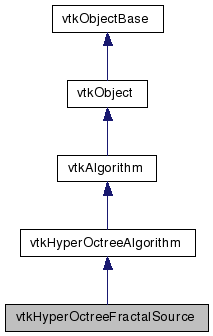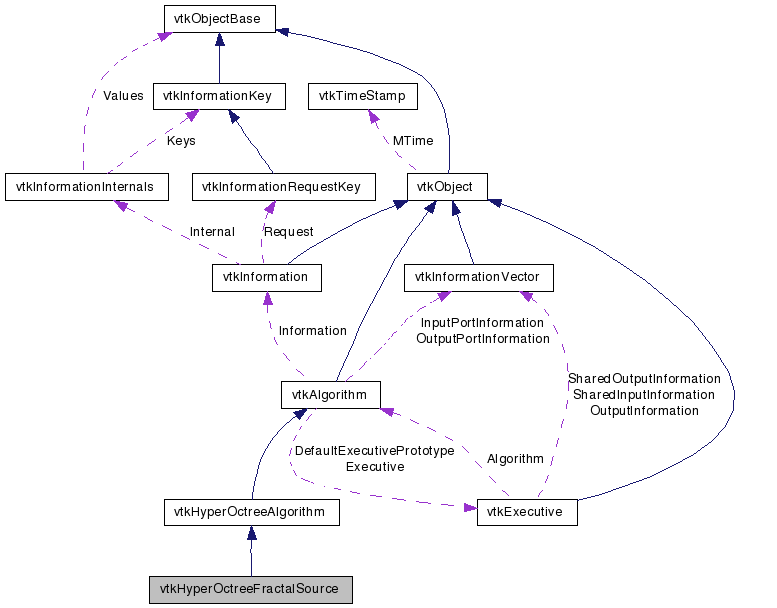vtkHyperOctreeFractalSource Class Reference

#include <vtkHyperOctreeFractalSource.h>

Inheritance diagram for vtkHyperOctreeFractalSource:[legend]
Collaboration diagram for vtkHyperOctreeFractalSource:[legend]

Detailed Description

Create an octree from a fractal. hyperoctree.

vtkHyperOctreeSampleFunction
Tests:
vtkHyperOctreeFractalSource (Tests)

Definition at line 34 of file vtkHyperOctreeFractalSource.h.

Public Types

typedef vtkHyperOctreeAlgorithm Superclass

Public Member Functions

virtual const char * GetClassName ()
virtual int IsA (const char *type)
void PrintSelf (ostream &os, vtkIndent indent)
int GetMaximumLevel ()
void SetMaximumLevel (int levels)
void SetMinimumLevel (int level)
int GetMinimumLevel ()
void SetProjectionAxes (int x, int y, int z)
void SetProjectionAxes (int a)
virtual int * GetProjectionAxes ()
virtual void GetProjectionAxes (int &, int &, int &)
virtual void GetProjectionAxes (int)
virtual void SetOriginCX (double, double, double, double)
virtual void SetOriginCX (double)
virtual double * GetOriginCX ()
virtual void GetOriginCX (double &, double &, double &, double &)
virtual void GetOriginCX (double)
virtual void SetSizeCX (double, double, double, double)
virtual void SetSizeCX (double)
virtual double * GetSizeCX ()
virtual void GetSizeCX (double &, double &, double &, double &)
virtual void GetSizeCX (double)
virtual void SetMaximumNumberOfIterations (unsigned short)
virtual unsigned char GetMaximumNumberOfIterations ()
virtual void SetDimension (int)
virtual int GetDimension ()
virtual void SetSpanThreshold (double)
virtual double GetSpanThreshold ()

Static Public Member Functions

static int IsTypeOf (const char *type)
static
vtkHyperOctreeFractalSource
SafeDownCast (vtkObject *o)
static
vtkHyperOctreeFractalSource
New ()

Protected Member Functions

vtkHyperOctreeFractalSource ()
~vtkHyperOctreeFractalSource ()
int RequestInformation (vtkInformation *vtkNotUsed(request), vtkInformationVector **vtkNotUsed(inputVector), vtkInformationVector *outputVector)
virtual int RequestData (vtkInformation *, vtkInformationVector **, vtkInformationVector *)
void Subdivide (vtkHyperOctreeCursor *cursor, int level, vtkHyperOctree *output, double *origin, double *size, float *cornerVals)
float EvaluateWorldPoint (double p)
float EvaluateSet (double p)

Protected Attributes

int MaximumLevel
int MinimumLevel
int Dimension
int ProjectionAxes 
unsigned char MaximumNumberOfIterations
double OriginCX 
double SizeCX 
double Origin 
double Size 
double SpanThreshold

Member Typedef Documentation

Reimplemented from vtkHyperOctreeAlgorithm.

Definition at line 37 of file vtkHyperOctreeFractalSource.h.

Constructor & Destructor Documentation

 vtkHyperOctreeFractalSource::vtkHyperOctreeFractalSource ( ) [protected]

 vtkHyperOctreeFractalSource::~vtkHyperOctreeFractalSource ( ) [protected]

Member Function Documentation

 virtual const char* vtkHyperOctreeFractalSource::GetClassName ( ) [virtual]

Reimplemented from vtkHyperOctreeAlgorithm.

 static int vtkHyperOctreeFractalSource::IsTypeOf ( const char * name ) [static]

Return 1 if this class type is the same type of (or a subclass of) the named class. Returns 0 otherwise. This method works in combination with vtkTypeRevisionMacro found in vtkSetGet.h.

Reimplemented from vtkHyperOctreeAlgorithm.

 virtual int vtkHyperOctreeFractalSource::IsA ( const char * name ) [virtual]

Return 1 if this class is the same type of (or a subclass of) the named class. Returns 0 otherwise. This method works in combination with vtkTypeRevisionMacro found in vtkSetGet.h.

Reimplemented from vtkHyperOctreeAlgorithm.

 static vtkHyperOctreeFractalSource* vtkHyperOctreeFractalSource::SafeDownCast ( vtkObject * o ) [static]

Reimplemented from vtkHyperOctreeAlgorithm.

 void vtkHyperOctreeFractalSource::PrintSelf ( ostream & os, vtkIndent indent ) [virtual]

Methods invoked by print to print information about the object including superclasses. Typically not called by the user (use Print() instead) but used in the hierarchical print process to combine the output of several classes.

Reimplemented from vtkHyperOctreeAlgorithm.

 static vtkHyperOctreeFractalSource* vtkHyperOctreeFractalSource::New ( ) [static]

Create an object with Debug turned off, modified time initialized to zero, and reference counting on.

Reimplemented from vtkAlgorithm.

 int vtkHyperOctreeFractalSource::GetMaximumLevel ( )

Return the maximum number of levels of the hyperoctree.

Postcondition:
positive_result: result>=1

 void vtkHyperOctreeFractalSource::SetMaximumLevel ( int levels )

Set the maximum number of levels of the hyperoctree. If GetMinLevels()>=levels, GetMinLevels() is changed to levels-1.

Precondition:
positive_levels: levels>=1
Postcondition:
is_set: this->GetLevels()==levels

min_is_valid: this->GetMinLevels()<this->GetLevels()

 void vtkHyperOctreeFractalSource::SetMinimumLevel ( int level )

Return the minimal number of levels of systematic subdivision.

Postcondition:
positive_result: result>=0

 int vtkHyperOctreeFractalSource::GetMinimumLevel ( )

Return the minimal number of levels of systematic subdivision.

Postcondition:
positive_result: result>=0

 void vtkHyperOctreeFractalSource::SetProjectionAxes ( int x, int y, int z )

Set the projection from the 4D space (4 parameters / 2 imaginary numbers) to the axes of the 3D Volume. 0=C_Real, 1=C_Imaginary, 2=X_Real, 4=X_Imaginary

 void vtkHyperOctreeFractalSource::SetProjectionAxes ( int a ) [inline]

Set the projection from the 4D space (4 parameters / 2 imaginary numbers) to the axes of the 3D Volume. 0=C_Real, 1=C_Imaginary, 2=X_Real, 4=X_Imaginary

Definition at line 67 of file vtkHyperOctreeFractalSource.h.

 virtual int* vtkHyperOctreeFractalSource::GetProjectionAxes ( ) [virtual]

Set the projection from the 4D space (4 parameters / 2 imaginary numbers) to the axes of the 3D Volume. 0=C_Real, 1=C_Imaginary, 2=X_Real, 4=X_Imaginary

 virtual void vtkHyperOctreeFractalSource::GetProjectionAxes ( int & , int & , int & ) [virtual]

Set the projection from the 4D space (4 parameters / 2 imaginary numbers) to the axes of the 3D Volume. 0=C_Real, 1=C_Imaginary, 2=X_Real, 4=X_Imaginary

 virtual void vtkHyperOctreeFractalSource::GetProjectionAxes ( int  ) [virtual]

Set the projection from the 4D space (4 parameters / 2 imaginary numbers) to the axes of the 3D Volume. 0=C_Real, 1=C_Imaginary, 2=X_Real, 4=X_Imaginary

 virtual void vtkHyperOctreeFractalSource::SetOriginCX ( double , double , double , double ) [virtual]

Imaginary and real value for C (constant in equation) and X (initial value).

 virtual void vtkHyperOctreeFractalSource::SetOriginCX ( double  ) [virtual]

Imaginary and real value for C (constant in equation) and X (initial value).

 virtual double* vtkHyperOctreeFractalSource::GetOriginCX ( ) [virtual]

Imaginary and real value for C (constant in equation) and X (initial value).

 virtual void vtkHyperOctreeFractalSource::GetOriginCX ( double & , double & , double & , double & ) [virtual]

Imaginary and real value for C (constant in equation) and X (initial value).

 virtual void vtkHyperOctreeFractalSource::GetOriginCX ( double  ) [virtual]

Imaginary and real value for C (constant in equation) and X (initial value).

 virtual void vtkHyperOctreeFractalSource::SetSizeCX ( double , double , double , double ) [virtual]

Just a different way of setting the sample. This sets the size of the 4D volume. SampleCX is computed from size and extent. Size is ignored when a dimension i 0 (collapsed).

 virtual void vtkHyperOctreeFractalSource::SetSizeCX ( double  ) [virtual]

Just a different way of setting the sample. This sets the size of the 4D volume. SampleCX is computed from size and extent. Size is ignored when a dimension i 0 (collapsed).

 virtual double* vtkHyperOctreeFractalSource::GetSizeCX ( ) [virtual]

Just a different way of setting the sample. This sets the size of the 4D volume. SampleCX is computed from size and extent. Size is ignored when a dimension i 0 (collapsed).

 virtual void vtkHyperOctreeFractalSource::GetSizeCX ( double & , double & , double & , double & ) [virtual]

Just a different way of setting the sample. This sets the size of the 4D volume. SampleCX is computed from size and extent. Size is ignored when a dimension i 0 (collapsed).

 virtual void vtkHyperOctreeFractalSource::GetSizeCX ( double  ) [virtual]

Just a different way of setting the sample. This sets the size of the 4D volume. SampleCX is computed from size and extent. Size is ignored when a dimension i 0 (collapsed).

 virtual void vtkHyperOctreeFractalSource::SetMaximumNumberOfIterations ( unsigned short ) [virtual]

The maximum number of cycles run to see if the value goes over 2

 virtual unsigned char vtkHyperOctreeFractalSource::GetMaximumNumberOfIterations ( ) [virtual]

The maximum number of cycles run to see if the value goes over 2

 virtual void vtkHyperOctreeFractalSource::SetDimension ( int ) [virtual]

Create a 2D or 3D fractal.

 virtual int vtkHyperOctreeFractalSource::GetDimension ( ) [virtual]

Create a 2D or 3D fractal.

 virtual void vtkHyperOctreeFractalSource::SetSpanThreshold ( double ) [virtual]

Controls when a leaf gets subdivided. If the corner values span a larger range than this value, the leaf is subdivided. This defaults to 2.

 virtual double vtkHyperOctreeFractalSource::GetSpanThreshold ( ) [virtual]

Controls when a leaf gets subdivided. If the corner values span a larger range than this value, the leaf is subdivided. This defaults to 2.

 int vtkHyperOctreeFractalSource::RequestInformation ( vtkInformation * vtkNotUsedrequest, vtkInformationVector ** vtkNotUsedinputVector, vtkInformationVector * outputVector ) [protected]

 virtual int vtkHyperOctreeFractalSource::RequestData ( vtkInformation * request, vtkInformationVector ** inputVector, vtkInformationVector * outputVector ) [protected, virtual]

This is called by the superclass. This is the method you should override.

Reimplemented from vtkHyperOctreeAlgorithm.

 void vtkHyperOctreeFractalSource::Subdivide ( vtkHyperOctreeCursor * cursor, int level, vtkHyperOctree * output, double * origin, double * size, float * cornerVals ) [protected]

 float vtkHyperOctreeFractalSource::EvaluateWorldPoint ( double p ) [protected]

 float vtkHyperOctreeFractalSource::EvaluateSet ( double p ) [protected]

Member Data Documentation

 int vtkHyperOctreeFractalSource::MaximumLevel [protected]

Definition at line 122 of file vtkHyperOctreeFractalSource.h.

 int vtkHyperOctreeFractalSource::MinimumLevel [protected]

Definition at line 123 of file vtkHyperOctreeFractalSource.h.

 int vtkHyperOctreeFractalSource::Dimension [protected]

Definition at line 124 of file vtkHyperOctreeFractalSource.h.

 int vtkHyperOctreeFractalSource::ProjectionAxes [protected]

Definition at line 126 of file vtkHyperOctreeFractalSource.h.

 unsigned char vtkHyperOctreeFractalSource::MaximumNumberOfIterations [protected]

Definition at line 128 of file vtkHyperOctreeFractalSource.h.

 double vtkHyperOctreeFractalSource::OriginCX [protected]

Definition at line 131 of file vtkHyperOctreeFractalSource.h.

 double vtkHyperOctreeFractalSource::SizeCX [protected]

Definition at line 135 of file vtkHyperOctreeFractalSource.h.

 double vtkHyperOctreeFractalSource::Origin [protected]

Definition at line 140 of file vtkHyperOctreeFractalSource.h.

 double vtkHyperOctreeFractalSource::Size [protected]

Definition at line 141 of file vtkHyperOctreeFractalSource.h.

 double vtkHyperOctreeFractalSource::SpanThreshold [protected]

Definition at line 143 of file vtkHyperOctreeFractalSource.h.

The documentation for this class was generated from the following file:

Generated on Wed Jun 3 19:07:51 2009 for VTK by1.5.6